# BINOM.DIST Function

Calculates the binomial distribution probability for the number of successes from a specified number of trials

## Calculate Binomial Distribution in Excel

The BINOM.DIST function is categorized under Excel Statistical functions. It calculates the binomial distribution probability for the number of successes from a specified number of trials. This binomial distribution Excel guide will show you how to use the function, step by step.

The binomial distribution is a statistical measure that is frequently used to indicate the probability of a specific number of successes occurring from a specific number of independent trials. The two forms used are:

• The Probability Mass Function – Calculates the probability of there being exactly x successes from n independent trials
• The Cumulative Distribution Function – Calculates the probability of there being at most x successes from n independent trials

In financial analysis, the BINOM.DIST function can be useful in finding out, for example, the probability of publishing a best-selling book from a range of books to be published by a company.

Introduced in Excel 2010, BINOM.DIST function is an updated version of the BINOMDIST function.

### Formula for Binomial Distribution

=BINOM.DIST(number_s,trials,probability_s,cumulative)

The BINOM.DIST uses the following arguments:

1. Number_s (required argument) This is the number of successes in trials.
2. Trials (required argument) This is the number of independent trials. It must be greater than or equal to 0.
3. Probability_s (required argument) This is the probability of success in each trial.
4. Cumulative (required argument) This is a logical value that determines the form of the function. It can either be:
1. TRUE – Uses the cumulative distribution function.
2. FALSE – Uses the probability mass function.

### How to use the Binomial Distribution Function in Excel?

To understand the uses of the BINOM.DIST function, let us consider an example:

#### Example

Suppose we are given the following data: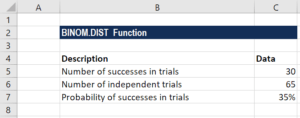The formula for calculating binomial distribution using the cumulative distribution function is shown below:

###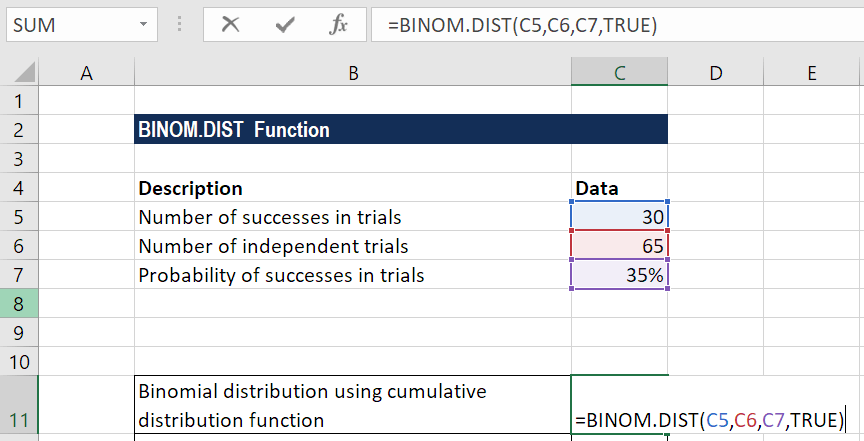We get the result below: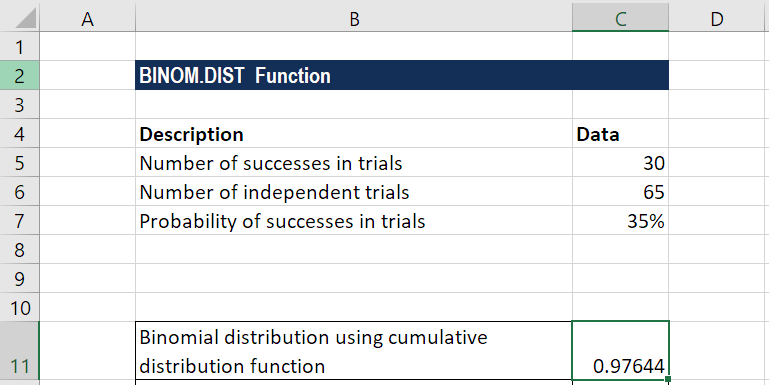The formula for calculating binomial distribution using the probability mass function is shown below: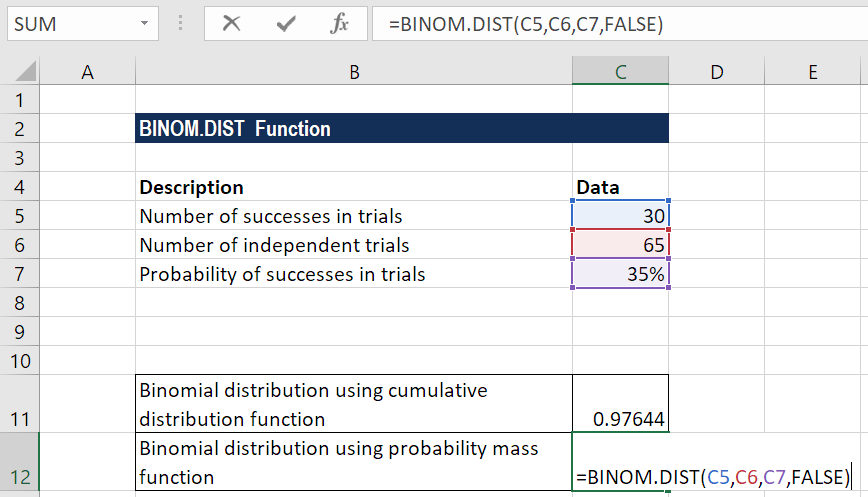We get the result below: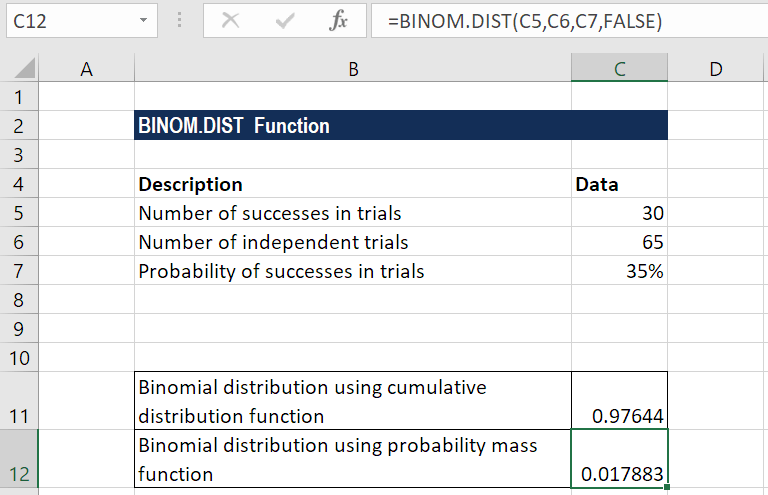### A few notes about the BINOM.DIST function

1. The BINOM.DIST function will truncate all numerical values to Integer.
2. #VALUE! error – Occurs when any of the arguments provided is non-numeric.
3. #NUM! error – Occurs when:
1. The given probability is less than zero or greater than 1.
2. The given number_s is less than zero or greater than the number of trials.

### Free Excel Course

If you want to learn more about Excel functions and become an expert on them, check out CFI’s Free Excel Crash Course! Go through our step by step instructions and demonstrations on how to become an Excel power user.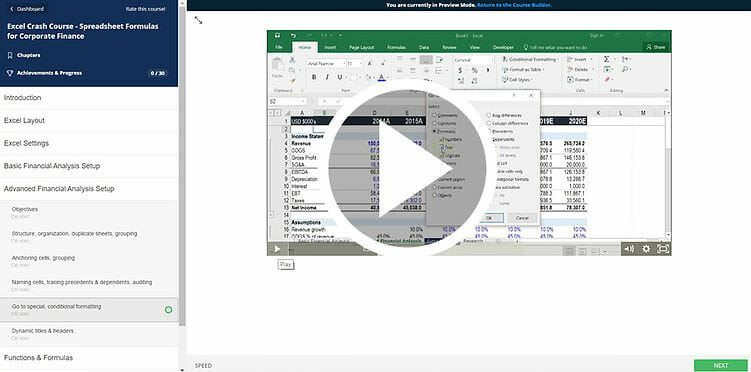Thanks for reading CFI’s guide to the binomial distribution function in Excel! By taking the time to learn and master these functions, you’ll significantly speed up your financial analysis. To learn more, check out these additional CFI resources:

• Excel Functions for Finance